Science Journal of Circuits, Systems and Signal Processing
Volume 4, Issue 1, February 2015, Pages: 1-8

Speech Enhancement Using Hilbert Spectrum and Wavelet Packet Based Soft-Thresholding

Md. Ekramul Hamid1, 2, Md. Khademul Islam Molla1, Md. Iqbal Aziz Khan1, 2, Takayoshi Nakai2

1Dept. of Computer Science and Engineering, University of Rajshahi, Rajshahi, Bangladesh

2Dept. of Electric and Electronic Engineering, Shizuoka University, Hamamatsu-shi, Japan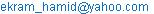(Md. E. Hamid)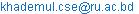(Md. K. I. Molla)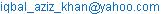(Md. I. A. Khan)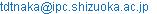(T. Nakai)

Md. Ekramul Hamid, Md. Khademul Islam Molla, Md. Iqbal Aziz Khan, Takayoshi Nakai. Speech Enhancement Using Hilbert Spectrum and Wavelet Packet Based Soft-Thresholding. Science Journal of Circuits, Systems and Signal Processing. Vol. 1, No. 1, 2015, pp. 1-8. doi: 10.11648/j.cssp.20150401.12

Abstract: A method of and a system for speech enhancement consists of Hilbert spectrum and wavelet packet analysis is studied. We implement ISA to separate speech and interfering signals from single mixture and wavelet packet based soft-thresholding algorithm to enhance the quality of target speech. The mixed signal is projected onto time-frequency (TF) space using empirical mode decomposition (EMD) based Hilbert spectrum (HS). Then a finite set of independent basis vectors are derived from the TF space by applying principal component analysis (PCA) and independent component analysis (ICA) sequentially. The vectors are clustered using hierarchical clustering to represent the independent subspaces corresponding to the component sources in the mixture. However, the speech quality of the separation algorithm is not enough and contains some residual noises. Therefore, in the next stage, the target speech is enhanced using wavelet packet decomposition (WPD) method where the speech activity is monitored by updating noise or unwanted signals statistics. The mode mixing issue of traditional EMD is addressed and resolved using ensemble EMD. The proposed algorithm is also tested using short-time Fourier transform (STFT) based spectrogram method. The simulation results show a noticeable performance in the field of audio source separation and speech enhancement.

Keywords: Speech Enhancement, Ensemble Empirical Mode Decomposition, Source Separation,
Independent Subspace Analysis, Hilbert Spectrum, Wavelet Packet Decomposition

1. Introduction

The problem of separating different sound sources can be classified as a denoising or enhancement problem, where the "signal" is the important part of the audio stream, and the "noise" is everything else. Although this is simple task for human auditory system, the automated audio source separation for speech enhancement can be considered as one of the most challenging topics in current research. Audio source separation or speech enhancement has many applications including robust automatic speech recognition, music transcription, surveillance applications, remixing of studio recording etc.

Speech quality may significantly deteriorate in the presence of interfering noise signals. The modern communications systems, such as cellular phones, employ some speech enhancement procedure at the preprocessing stage, prior to further processing . One approach to separate the mixed audio signals is microphone array processing . The array processing requires hug computation and inefficient to be used in real world applications. Hence, present research trend is to reduce the number of microphones used in recording of the intended acoustical environment. Several noise reduction schemes have been developed which try to suppress the signal components corresponding to noise and enhance the target component. This technique corresponds to the use of only one microphone. For instance, in the application of spectral noise suppression schemes [3,4,5] to speech enhancement it is assumed that the signal of interest is the speech with its typical speech pauses while the noise signal is regarded as stationary and uninterrupted. Therefore, it is possible to estimate the noise spectrum during speech pauses and subsequently subtract it from the spectrum of the noise contaminated speech segments in order to obtain the enhanced speech signal. Computational Auditory Scene Analysis (CASA) is one of the first methods that tried to decrypt the human auditory system in order to perform an automatic audio source separation system . A recent advancement of single mixture audio separation is the independent subspace analysis (ISA) method [7,8]. The study  describes a single stage source separation using EMD and ICA. The method proposed KLD based clustering algorithm to group the independent basis vectors and experimental results show a good source separation performance. The implementation of ISA is the extension of basic ICA by decomposing an audio mixture into independent source subspaces. Westner  implemented ISA method to decompose a mixture spectrogram into independent source subspaces and inverting them to yield source separation. They employed short-time Fourier transformation (STFT) to produce the time-frequency (TF) representation (spectrogram) of the mixed signal and derived a set of frequency-independent basis vectors to represent the source subspaces. The STFT employed in TF representation includes a certain amount of cross-spectral energy during the overlap of the window between the successive time frames. Two major limitations of STFT degrade the disjoint orthogonality of the audio sources in TF domain. Another study by Ghanbariet all in  presents a speech enhancement algorithm based on adaptive thresholding wavelet packet decomposition. A new VAD is designed in wavelet domain and the method shows high performance in speech enhancement.

The proposed method of speech enhancement consists of two stages processing. In the first stage a source separation algorithm is implemented using Hilbert spectrum where ISA is used to separate audio sources from a single mixture and in the second stage, a adaptive denoising algorithm is implemented based on wavelet packet decomposition which further enhance the target speech quality. The Hilbert spectrum (HS) is employed for the TF representation of the audio signals. The HS does not include noticeable amount of cross-spectral energy terms. It is able to represent the instantaneous spectral information of any time series without windowing. The mode mixing issue of traditional EMD  is addressed and resolved using ensemble EMD and Hilbert transformation are employed together to derive HS. The decomposition of the mixed signal in spectral domain is obtained by the spectral projection between the mixture and each IMF component. This vector space is used to derive a set of independent basis vectors by applying PCA and ICA. The hierarchical clustering algorithm is used to group the basis vectors into the given number of component sources. A further removal of background noise is obtained in the second stage by wavelet packet decomposition (WPD). The enhancement process consists of a simple voice activity detection (VAD) followed by noise estimation on the basis of calculated subband SNR. From these values an adaptive soft thresholding parameter is derived to update the wavelet coefficients. This technique works well in cascade with the source separation method. The waveforms, ISNR as well as OSSR values show that this new multistage technique highly improves the performance of speech enhancement results.

Regarding the organization of this paper, the basics of EMD and HS are described in section 2, the separation and enhancement algorithm are described in section 3 and 4, the experimental results are presented in section 5, and finally Section 6 contains conclusion of the study.

2. EMD Based Hilbert Spectrum (HS)

The principle of the EMD technique is to decompose any signal x(t) into a set intrinsic mode functions Cm(t) (IMFs). Each IMF satisfies two basic conditions: in the whole data set the number of extrema and the number of zero crossing must be same or differ at most by one, (ii) at any point, the mean value of the envelope defined by the local maxima and the envelope defined by the local minima is zero. At the end of the decomposition the signal x(t) is represented as: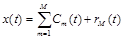(1)

where M is the number of IMFs and rM(t) is the final residue. Due to the presence of noise, traditional EMD is survived by mode mixing problem. Mode mixing problem is defined as an IMF that includes oscillations of dramatically disparate scales or a component of similar scale residing in different IMFs . This issue is resolved by introducing ensemble EMD algorithm.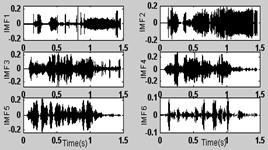Figure 1. IMFs of an audio mixture.First six IMFs out of total 16 IMFs.

2.1.Instantaneous Frequency

Instantaneous frequency (IF) is defined as the rate of change of the phase. Every IMF is a real valued signal. The discrete Hilbert transform (HT) is used to compute the analytic signal for an IMF. The discrete HT denoted by Hd[.]of the signal x(t)is given by: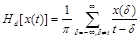(2)

Then the analytic version of the mth IMF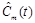is defined as: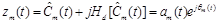(3)

where am(t) and qm(t) are instantaneous amplitude and phase respectively of the mth IMF. The analytic signal can be used in determining the instantaneous quantities such as energy, phase and frequency. The discrete-time IF of mth IMF is then given as the derivative of the phase qm(t) i.e.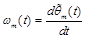(4)

where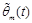represents the unwrapped version of instantaneous phase qm(t).

2.2. Hilbert Spectrum

Hilbert Spectrum represents the distribution of the signal energy as a function of time and frequency. Hilbert transform on each IMF, the time series data can be expressed as: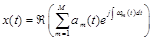(5)

where Â(.) represents the real part of the complex number and only M number of IMFs are taken into consideration leaving the residue. To construct the Hilbert spectrum, the IF values are first normalized between 0 to 0.5, then each IF vector is multiplied by a scaling factor h .

The overall HS H(n,t) is defined as the weighted sum of the instantaneous amplitudes of all the IMFs at nth frequency bin.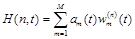(6)

where the weight factor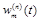takes 1 if h×wm(t) falls within nth band, otherwise is 0. After computing the elements over the frequency bins, H represents the instantaneous signal spectrum in TF space as a 2D table shown in figure 2.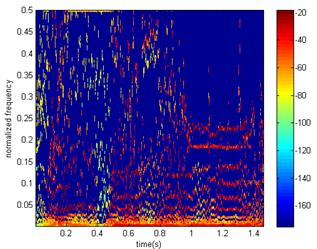Figure 2. Hilbert spectrum (HS) of an audio mixture using 256 frequency bins.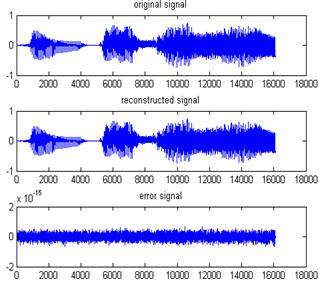Figure 3. The original signal (top), reconstructed signal (middle) and the error signal (bottom).

2.3. Signal Reconstruction from HS

With step-by-step summing up the IMFs, it is possible to build up the original signal with a minimum error. During the Hilbert transform the real part of the signal remains unchanged. The time domain signal xr(t) is easily be reconstructed by filtering out the imaginary part from the HS and summing over frequency bins as: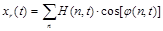(7)

where f(n,t) is the phase matrix of Hilbert spectrum. The phase matrix is saved during the construction of Hilbert spectrum to be used in re-synthesis. The signal xr(t) is reconstructed from the Hilbert spectrum given in figure 3. The error between the original signal x(t) and xr(t) is also shown.

3. Subspace Decomposition of Hilbert Spectrum

The single mixture blind source separation BSS technique decomposes the TF space of the mixture as the sum of independent source subspaces. The ensemble empirical mode decomposition (EMD) and Hilbert transformation are employed together to derive HS. The Hilbert Spectrum of the mixture signal is constructed by properly arranging the frequency responses of the individual IMF along time and frequency axes with preferred number of frequency bins.

3.1. Algorithm for Source Separation

The block diagram of the overall enhancement technique is shown in figure 4. In this work, only the mixture of two audio sources are taken into account and hence k=2. One source is the speech signal and the other corresponds to the interfering signal, which can be any noise source. The proposed single mixture source separation algorithm is based on decomposing the Hilbert spectrum H of mixture signal into a number of subspaces corresponding to each independent source. It is assumed that the H can be expressed as the weighted sum of p independent basis vectors fi. The Hilbert spectrum can be represented in terms of temporal basis vector zi as: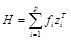(8)

where T stands here as transpose operator, each fi and zi is a column vector of length equal to the number of frequency bins and the number of time slices in H, respectively. The subspaces are composed of a matrix with spectral independent basis vectors in the columns as,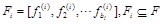, where bi is the required number of basis vectors to represent the ith subspace. The time-varying weight coefficients, i.e. temporal basis vectors can be obtained from matrix multiplication of the pseudo-inverse of the basis vectors spanned by Fi with H as: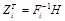,whereZi is the matrix containing the temporal coefficients corresponding to Fi, and is represented as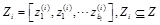. The Hilbert spectrum H of the mixture signal can be decomposed as the sums of k independent source subspaces,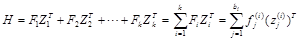(9)

The principal objective of the proposed separation method is to derive some independent basis vectors and to group them into k subsets (Fi; i=1, 2, …, k) representing the spectra of the individual audio sources.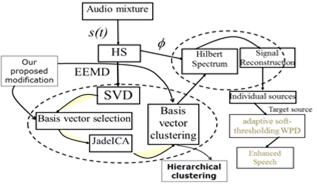Figure 4. Block diagram of the enhancement algorithm using proposed technique.

3.2. Basis Vector Selection

Singular value decomposition (SVD) is a well-definedgeneralization of the PCA and becomes an important tool in statistical data analysis and signal processing. SVD of Xn´l is the factorization of the form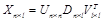, where U and V are orthogonal matrices (with orthogonal columns) and D is a diagonal matrix of M singular values.   The spectral projection (coherence) vectors of the mixture on to individual IMF components are used to derive the spectral independent basis vectors. The spectral projection of the mixture signal x(t)on to the mth IMF is defined as: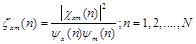(10)

where cxm(n) is the cross spectrum of the mixture and the mth IMF, yx(n) and ym(n) are the marginal power spectra of the mixture signal and the mth IMF respectively at frequency index n. The term zxm(n) is a quantitative measure of how much the mixture is correlated with mth IMF at nth frequency bin.

3.3. Independent Basis Vectors

The basis vectors obtained by SVD are not statistically independent but they are uncorrelated. The selected basis vectors uiÎU or viÎV will undergo the ICA process to derive the independent source subspaces. ICA yields the observation vector g as the product of mixing matrix G and the vectors of statistically independent signals as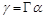, where G is an q´r (pseudo-) invertible mixing matrix with orthogonal columns, a is the random vector of r signals, and g is the q-dimensional vector of observations with q³r .  In this model, g is the reduced set of basis vectors, i.e.,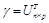if selected from U or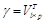if selected from V, and p is the number of required basis vectors determined by the dimension reduction method. The principle of the ICA algorithm is to determine the recovery (unmixing) matrix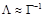such that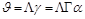(11)

where J is the vector containing the statistically independent signals which are very similar to a.  The JadeICA algorithm  is used here to estimate matrix L from the reduced set of selected basis vectors. The resulting vector J corresponds to the spectral-independent basis vector FT=[f1, f2, f3……fp]or temporal-independent basis vector ZT=[z1, z2, z3……zp] depending on the selection of g from U or V, respectively.

3.4. Clustering of Independent Basis Vectors

Once the spectral independent basis vectors are obtained, the basis vectors are then grouped into the number of sources. The proposed hierarchical clustering algorithm for finding the clusters around the mixing vectors is tested. We follow bottom-up (agglomerative) strategy that the starting point is the single samples, considering them as clusters that contain only one object. Clusters are then combined, so that the number of clusters decreases while the average number of objects per cluster increases. The clustering algorithm to gather the independent basis vectors into k groups (here, k=2). To measure the distance between clusters, we use the Euclidean distance based on the normalized samples. Given a set of N items to clustered and an N*N distance (or similarity) matrix and D=[d(i,j)] is the proximity matrix. The algorithm is summarized bellow :

1)         Start with the disjoint clustering having level L(0) = 0 and sequence number m=0.

2)         Find the least dissimilar pair (r), (s) of clusters in the current clustering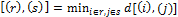, where the minimum is over all pairs of clusters in the current clustering.

3)         Increment the sequence number: m=m+1. Merge clusters (r) and (s) into a single cluster to form next clustering m. Set the level of this clustering to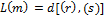.

4)         Update the proximity matrix, D, by deleting the rows and columns corresponding to clusters (r) and (s) and adding new rows and columns to the new formed cluster (k). Then the proximity between the new and old cluster is defined as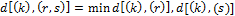if all objects are in one cluster, stop. Otherwise, go to step 2.

We have implemented a reversible process from Hilbert spectrum to get back the time domain target speech signal as discussed in the previous section 2.3.

4. Speech Enhancement using Wavelet Packet Decomposition (WPD)

The wavelet packet transform (WPT) is a generalization of the decomposition process that offers a better performance compared to the ordinary wavelet methods . In the wavelet analysis, a signal is split into an approximation and a detail. The approximation is then itself split into a second-level approximation and detail and the process is repeated. By applying WPT on each input frame we produce 2Jsubband wavelet packets, where J is the number of levels for WPT. If W denotes the wavelet transform matrix, then the separated speech y(t) after the first stage in wavelet domain is defined as Y=Speech+Noise. The proposed enhancement system consists of the following sections:

4.1. SNR Estimation

We calculate signal-to-noise ratio (SNR) for each subband which provides information about the noise in the current subband of wavelet packets using the relation: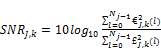(12)

where is the wavelet packet coefficients in the level J of the k-th subband and £ is the estimated noise. The estimated noise £ is updated during the silence segment as detected by the Voice Activity Detector (VAD). We have used a simple VAD based on adaptive linear energy measures . For that a full-band energy measure calculates the wavelet packets energy of the incoming frames. Then the threshold value is calculated to estimate the background noise. The initial threshold is defined as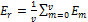, where v is the number of frames and Em is energy of m-th frame. Now if the frame of the incoming frame is greater than the threshold, the frame is judged as a speech frame. The new threshold is calculated as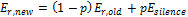, where Esilence is the energy calculated from the silence frames which is calculated from the variance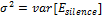of the observed speech. The coefficient p (0<p<1) is taken adaptively from the ratio of the variance of the incoming frame and variance of the silence frame.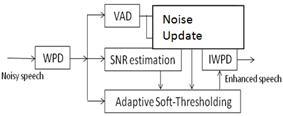Figure 5. Block diagram of the speech enhancement method in stage-2 using WPD based soft-thresholding.

4.2. Noise Update

During the silence segments, we update the estimated subband noise energy as: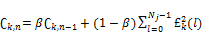(13)

wheredenotes the k-th subband energy of n-th silence segment and coefficient β is 0.9 which is selected by experiment.

We estimate node wise threshold to handle non-stationary noise segment present in the separated target speech.  When the input frame is detected as a silence frame, the node depended threshold A is updated. Then we update the threshold adaptively on the basis of the SNR for each subband is defined as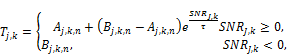(14)

where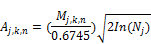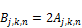and n is the index of the silence segments, M represents the median absolute estimated on the scale j and subband k and A represents the estimated threshold value of the scale j and the subband k updated at the n-th detected silence segment.  The factor τ is a factor to make the threshold approximately equal to Aj,k,n. The factor 2< τ seems to be adequate so that for SNR>10dB where the threshold values equal to Aj,k,n. Then the proposed non linear soft-thresholding function is determined as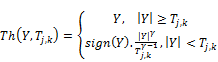(15)

where γ=3 which is used for optimization and Y in the target speech in wavelet domain. This value is used for thresholding the wavelet packet coefficients of the separated target speech in each subband .

5. Experimental Results

The efficiency of the proposed enhancement technique is tested in two steps, 1) separate the signals from the mixture of two audio sources and 2) enhance the quality of the target source. Both mix1 (speech with telephone ring sound) and mix2 (speech with flute sound) mixtures are used in the experiments.  The two recorded signals with normalized amplitudes are added to make 0dB SNRs.  The speech signal of each mixture comprises utterances of several words spoken by the same speaker. The audio signals are sampled at 16 kHz sampling rate and 16-bit amplitude resolution. The mixed signal is divided into blocks of 0.25s by using Tukey window with 50% overlapping. The average value of the running short-term relative energy between the original and separated signals is used to measure the separation efficiency is termed as the original-to-separated-signal ratio (OSSR) and defined mathematically as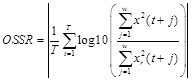(16)

where x(t) and xr(t) are the original and separated (reconstructed) signals, respectively, T is the total time length, w is a 10ms square window. The OSSR values show the similarity between two signals. If the two signals are exactly equal, the OSSR value will be 0, that is a smaller deviation of OSSR from 0 indicates a higher degree of separation. Henceforth, the male speech is denoted as Signal1 and instrument signals as Signal2.

Table 1 shows the average OSSR of each signal for both mixtures. The separation efficiency is compared between the Hilbert-based method and the Fourier-based one; it shows that the performance is higher for the Hilbert-based method.

Table 1. Experimental results (in terms of OSSR) of the proposed separation algorithm using Fourier and Hilbert based methods.

 Mixtures OSSR of Signal1 OSSR of Signal2 Fourier Hilbert Fourier Hilbert mix1 (speech+ring) 0.37 0.26 0.04 0.02 mix2 (speech+flute) 0.44 0.36 0.25 0.14

The degradation of separation performance using Fourier-based is increased due to overlapping in spectrum results a lower TF resolution. Figure 6 and figure 7 show the separation and enhancement results of speech flute mixture using Fourier-based method. Figure 8 shows the same experimental result of speech ring mixture using Hilbert-based method.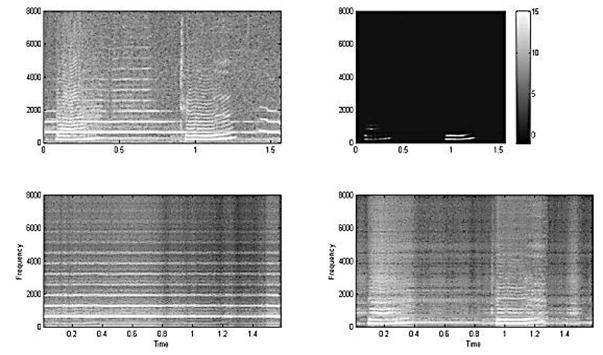Figure 6. Spectrograms: mixed signal (TL), Separated signals -flute (BL), speech (BR), Error (TR) using Fourier-based method.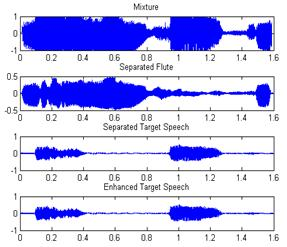Figure 7. Waveforms (from top): mixed signal, Separated signals -flute, speech and enhanced target speech using Fourier-based method.

To study the quantitative analysis of enhancement performance in two stages, we have employed an improvement of signal to noise ratio (ISNR) measure. The ISNR [ISNR (dB)=SNRin-SNRout] represents the degree of enhancement of the target signal when it is degraded by interfering noise.  Here SNRinand SNRout represent the input and output SNRs, respectively. Table 2 shows the ISNR of target signal for three mixtures using Fourier and Hilbert based methods in two stages. The higher value of ISNR indicates better quality signal and it is observed that Hilbert based method is better than Fourier based method (in stage 1) and a further improvement is obtained after WPD based soft thresholding method (in stage 2). It is observed that the separated speech from stage-1 contains some residual noise which is successfully reduced in stage-2.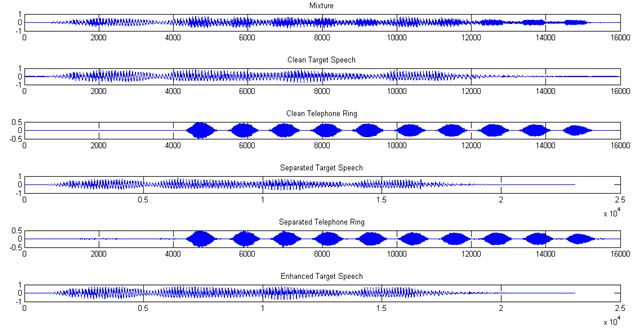Figure 8. Waveforms (from top): Mixed signal, Clean signal- speech and ring tone, Separated signal- speech and ring tone and lastly the enhanced target speech using Hilbert-based method.

Table 2. Experimental results (in terms of ISNR) of the proposed two stages enhancement algorithm using Fourier and Hilbert based methods in two stages.

 Mixture Method signal1 Stage-1 (ISNR) Stage-2 (ISNR) mix1 (speech+ring) Fourier 7.2 7.5 Hilbert 8.9 9.5 mix2 (speech+flute) Fourier 6.8 7.0 Hilbert 8.0 8.8 mix3 (speech+white     noise) Fourier 6.0 7.4 Hilbert 8.1 9.2

6. Conclusion

We have presented a two stage method for speech enhancement. It describes the effectiveness of Hilbert spectrum (HS) in time-frequency (TF) representation of audio signals. The efficiency of HS has been compared with short-time Fourier transform (STFT) as a method of TF representation with the consideration of disjoint orthogonality of audio signals and the experimental results show that Hilbert spectrum performs better than STFT based spectrogram for TF representation. Usually, the higher resolution in TF representation demonstrates the signal in more detail and hence improves the separation performance. A set of independent basis vectors are derived from HS by applying principal component analysis (PCA) and ICA in sequence.  In both algorithms, hierarchal clustering is employed to group the independent bases to derive the source subspaces.  The experiments show that the use of Hilbert spectrum in time-frequency representation fits better in subspace decomposition than Fourier-based method does and it obviously increases the separation efficiency. The distortion due to residual noise in the target speech is handled at the second stage using wavelet packet based soft-thresholding method which made considerable enhancement. The approach uses a wavelet signal processing strategy and controls the threshold values based on estimated subband SNRs to remove noise components that exists after the separation algorithm. The simulation results show a noticeable performance in the field of audio source separation and speech enhancement. A further improvement is expected by implementing the whole process in wavelet packet domain instead of EMD. The improvement of the robustness of the separation process will be addressed in the future work.

Acknowledgement

We would like to thank to National Institute of Information and Communications Technology (NICT) for providing support to carry out the study.

References

1. H. Saruwatari, S. Kurita, K. Takeda, F. Itakura, T. Nishikawa, and K. Shikano, "Blind Source Separation Combining Independent Component Analysis and Beamforming." EURASIP Journal on Applied Signal Processing, vol. 11, pp. 1135-1146, 2003.
2. J. M. Valin, J. Rouat, and F. Michaud, "Enhanced Robot Audition Based on Microphone Array Source Separation with Post-Filter," Proc. IEEE/RSJ International Conference on Intelligent Robots and Systems (IROS), 2004.
3. Y. Ephraim, and D. Malah, "Speech enhancement using a minimum mean-square error short-time spectral amplitude estimator," IEEE Trans. on Acoustic, Speech and Signals Processing, vol. 32, pp. 1109-1121, 1984.
4. O. Cappe, "Estimation of the musical noise phenomenon with the Ephraim and Malah noise suppressor," IEEE Trans. on Acoustic, Speech and Signals Processing, vol. 2, pp. 345-349, 1994.
5. S. F. Boll, "Suppression of acoustic noise in speech using spectral subtraction," IEEE Trans. on Acoustic, Speech and Signals Processing, vol. 27, pp. 113-120, 1979.
6. G. J. Brown, and M. Cooke,"Computational auditory scene analysis," Computer Speech Language, vol. 8(4), pp. 297-336, 1994.
7. M. A.Casey, and A. Westner, "Separation of mixed audio sources by independent subspace analysis," Proc. of International Computer Music Conference, pp. 154-161, 2000.
8. M. K. I. Molla, and K. Hirose, "Single mixture audio source separation by subspace decomposition of Hilbert spectrum," IEEE transactions on audio, speech and language processing, vol. 15(3), pp. 893-900, 2007.
9. Y. Ghanbari, and M. R. K. Mollaei, "A new approach for speech enhancement based on the adaptive thresholding of the wavelet packets", Speech Communications, Elsevier,  vol. 48, pp. 927-940, 2006.
10. N. E. Huang, Z.Shen, S. R Long, et al. "The empirical mode decomposition and Hilbert spectrum for nonlinear and non-stationary time series analysis," Proc. Roy. Soc. London A, vol. 454, pp. 903-995, 1998.
11. Z. Wu, and N. E. Huang, "Ensemble empirical mode decomposition: a noise-assisted data analysis method," Advances in Adaptive Data Analysis, vol. 1(1), 2009.
12. A. Hyvärinen,and E. Oja, "Independent component analysis: algorithms and applications,"Neural Networks, vol.13(4-5), pp. 411-430, 2000.
13. J. F.Cardoso,and A. Souloumiac, "Blind beamforming for nongaussian signals," IEE Proceedings-F,pp. 362-370, 1993.
14. J. Rosca, D.Erdogmus, J. Princip, and S. Haykin, Independent component analysis and blind signal separation, Springer, 2006.
15. R. A. Singer, R. G. Sea, "A new filter for optimal tracking in dense multi-target environment," Proceedings of the ninth Allerton Conference Circuit and System Theory. Urbana-Champaign, USA: Univ. of Illinois, pp. 201-211,1971.
16. N. E. Huang, et al.,"Application of Hilbert-Huang transform to non-stationary financial time series analysis," Applied Stochastic Model in Business and Industry, vol. 19, pp. 245-268, 2003.

 Contents 1. 2. 2.1. 2.2. 2.3. 3. 3.1. 3.2. 3.3. 3.4. 4. 4.1. 4.2. 4.3. 5. 6.
Article ToolsAbstractPDF(673K)